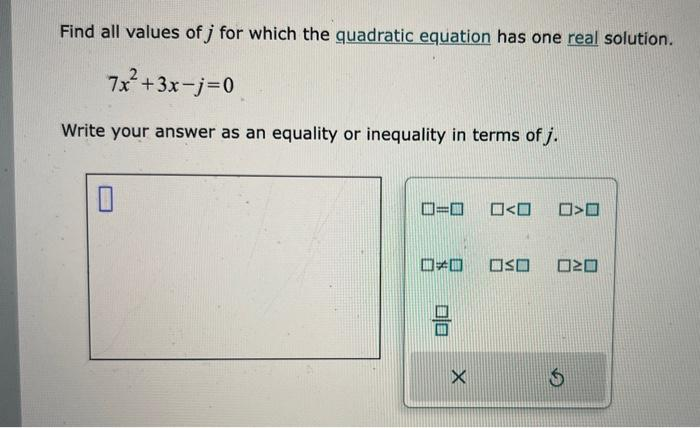# (Solved): Find all values of j for which the quadratic equation has one real solution. 7x2+3xj=0 Write you ...Find all values of for which the quadratic equation has one real solution. Write your answer as an equality or inequality in terms of .

We have an Answer from Expert